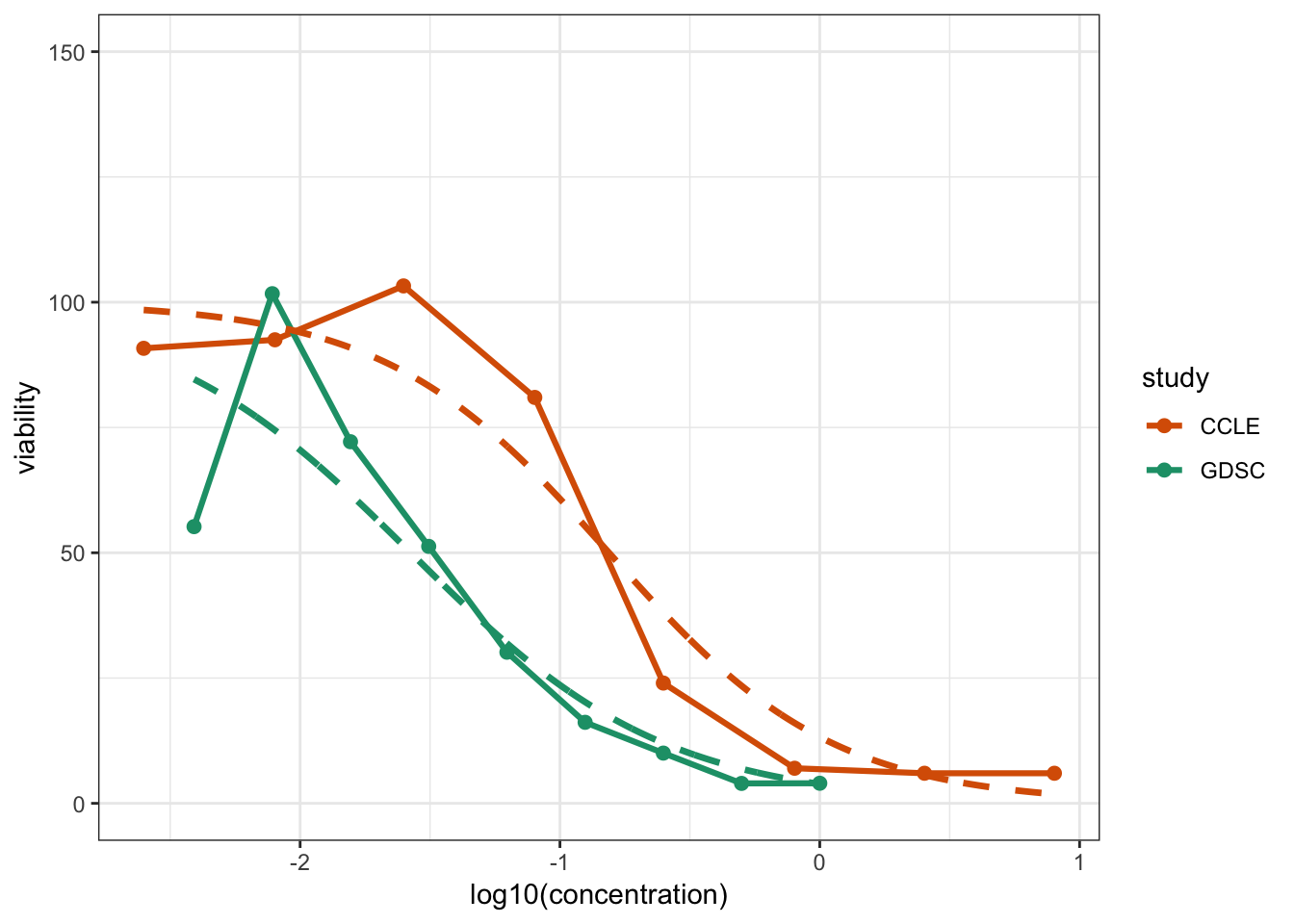## Introduction

In this supplementary tutorial we will take a look at calculating the AUC and IC50 metrics based by fitting curves to the observed viability scores and drug concentrations in the raw pharmacological data. We will take a look at doing this using logisitic regression, and more briefly, using linear regression.

The computed AUC and IC50 values are stored in a `RDS` file, `modelSummarizedPharmacoData.rds`, and explored in Tutorial 2b, “Digging Deeper with Drug Response Summarization”. This tutorial walks through how those values were computed.

## Setup Workspace

We start by loading the tidyverse family of packages and specifying a default plotting theme for our `ggplot` graphics.

``library(tidyverse)``
``````## Registered S3 methods overwritten by 'ggplot2':
##   method         from
##   [.quosures     rlang
##   c.quosures     rlang
##   print.quosures rlang``````
``## ── Attaching packages ────────────────────────────────── tidyverse 1.2.1 ──``
``````## ✔ ggplot2 3.1.1     ✔ purrr   0.3.2
## ✔ tibble  2.1.2     ✔ dplyr   0.8.1
## ✔ tidyr   0.8.3     ✔ stringr 1.4.0
## ✔ readr   1.3.1     ✔ forcats 0.4.0``````
``````## ── Conflicts ───────────────────────────────────── tidyverse_conflicts() ──
``theme_set(theme_bw())``

We will be using both the raw and summarized pharmacological data in this tutorial.

``````pharmacoData <- readRDS(file.path("..", "data", "rawPharmacoData.rds"))

## Logistic Regression

A common way to model viability response curves is to fit logistic regression models. If you have interest in knowing more about either logistic regression models or modeling approaches in general, this book gives an excellent introduction to these topics.

The idea of a drug response model is that it should describe how the viability changes with as a function of the drug concentration.

Let’s write a function that fits a logistic regression model on the data. The `fitLogisticModel` function defined below receives as input a drug, a cell line and a study, and fits a regression model, namely `viability ~ concentration` as described above, on these data.

``````fitLogisticModel <- function(drugA, cellLineA, studyA){
pharSub <- filter( pharmacoData, drug==drugA, cellLine==cellLineA, study==studyA)
inRange <- pharSub\$viability > 0 & pharSub\$viability < 100
pharSub\$viability <- round(pharSub\$viability)
pharSub\$concentration <- log10( pharSub\$concentration )
maxVal <- pmax( pharSub\$viability, 100 )
fit <- glm( cbind( viability, maxVal-viability ) ~ concentration,
pharSub, family=binomial )
fit
}``````

Let’s now use this function to fit models on the data. We will use the two drug-cell line combinations mentioned in the first section of this vignette.

``````lrCCLE1 <- fitLogisticModel( "17-AAG", "H4", "CCLE" )
lrGDSC1 <- fitLogisticModel( "17-AAG", "H4", "GDSC" )

lrCCLE2 <- fitLogisticModel( "Nilotinib", "22RV1", "CCLE" )
lrGDSC2 <- fitLogisticModel( "Nilotinib", "22RV1", "GDSC" )

lrCCLE1``````
``````##
## Call:  glm(formula = cbind(viability, maxVal - viability) ~ concentration,
##     family = binomial, data = pharSub)
##
## Coefficients:
##   (Intercept)  concentration
##        -1.880         -2.317
##
## Degrees of Freedom: 7 Total (i.e. Null);  6 Residual
## Null Deviance:       647.6
## Residual Deviance: 83.29     AIC: 115.4``````
``lrCCLE2``
``````##
## Call:  glm(formula = cbind(viability, maxVal - viability) ~ concentration,
##     family = binomial, data = pharSub)
##
## Coefficients:
##   (Intercept)  concentration
##         4.142         -2.104
##
## Degrees of Freedom: 7 Total (i.e. Null);  6 Residual
## Null Deviance:       59.92
## Residual Deviance: 25.39     AIC: 36.32``````

Let’s evaluate the logistic regression models by plotting the model and the raw data together. The function predictValues receives as input a fit and outputs response values predicted from such model. The plotFit function defined below enables the visualization of the model predictions together with the raw data.

``````predictValues <- function(fit, numPred = 1000) {
min <- min( fit\$data\$concentration )
max <- max( fit\$data\$concentration )
valuesToPredict <- seq(min, max, length.out=numPred)
predicted <- predict( fit,
data.frame(concentration=valuesToPredict),
type="response" )
data.frame( concentration=valuesToPredict,
viability=predicted*100 )
}

plotFit <- function(drugA, cellLineA, fitCCLE, fitGDSC) {
pharSub <- filter(pharmacoData, drug == drugA, cellLine == cellLineA)
sumSub <- filter(summarizedData, drug == drugA, cellLine == cellLineA)
p <- ggplot(pharSub, aes(x = log10(concentration), y = viability, color = study)) +
geom_point(size = 2.1) +
geom_line(lwd = 1.1) +
ylim(0, 150) +
scale_colour_manual(values = c("CCLE" = "#d95f02", "GDSC" = "#1b9e77")) +
xlim(range(log10(c(pharSub\$concentration, sumSub\$ic50_CCLE, sumSub\$ic50_GDSC))))
p <- p +
geom_line(aes(concentration, viability),
data = predictValues(fitCCLE), lwd = 1.2,
linetype = "dashed", color = "#d95f02") +
geom_line(aes(concentration, viability),
data = predictValues(fitGDSC), lwd = 1.2,
linetype = "dashed", col = "#1b9e77")
p
}``````

Now let’s use these functions to evaluate the regression fits from the two drug-cell line combinations mentioned before. Ideally, we would like the regression model to be as close as possible to the individual data points.

``plotFit("17-AAG", "H4", fitCCLE = lrCCLE1, fitGDSC=lrGDSC1 )````````plotFit("Nilotinib", "22RV1", fitCCLE = lrCCLE2, fitGDSC = lrGDSC2) +
xlim(-2, 1.3)``````
``````## Scale for 'x' is already present. Adding another scale for 'x', which
## will replace the existing scale.``````